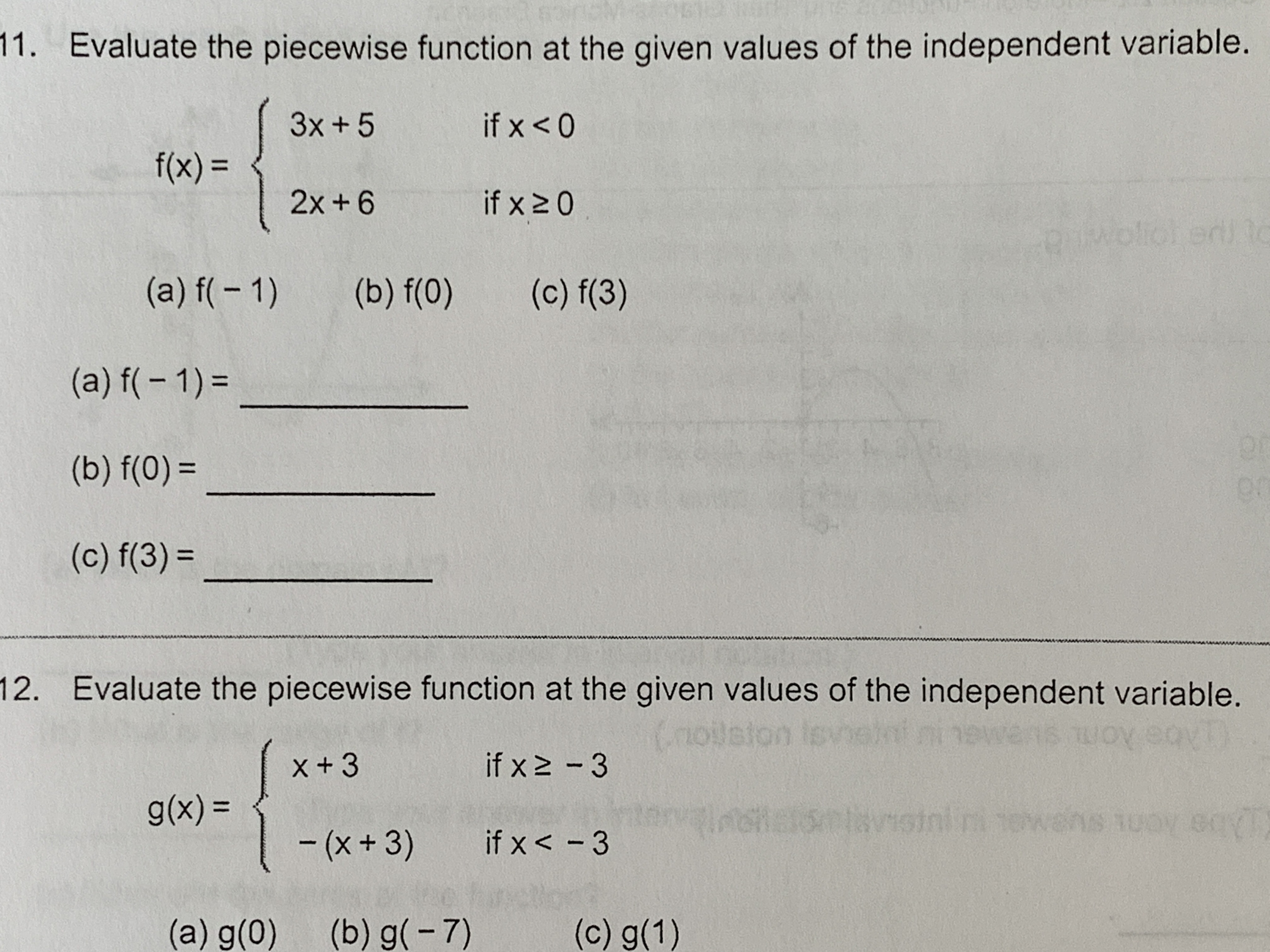11. Evaluate the piecewise function at the given values of the independent variable.3x+ 5if x < 0f(x)=2x + 6if x 2 0ed)(a) f( 1)(b) f(0)(c) f(3)(a) f( 1)(b) f(0)=Qr(c) f(3)=12. Evaluate the piecewise function at the given values of the independent variable.uosssisoronaiontavisininX+ 3if x 2 3Abe Aom simaug(x) =10nns ot-(x+ 3)if x< -3(a) g(0)(b) g(-7)(c) g(1)

Question

Can someone help me solve this problem? It ia the first one number elevenhelp_outlineImage Transcriptionclose11. Evaluate the piecewise function at the given values of the independent variable. 3x+ 5 if x < 0 f(x)= 2x + 6 if x 2 0 ed) (a) f( 1) (b) f(0) (c) f(3) (a) f( 1) (b) f(0)= Qr (c) f(3)= 12. Evaluate the piecewise function at the given values of the independent variable. uosssiso ronaiontavisinin X+ 3 if x 2 3 Abe Aom simau g(x) = 10n ns ot -(x+ 3) if x< -3 (a) g(0) (b) g(-7) (c) g(1) fullscreen
Step 1

To find the values of f at the prescribed points , ...

Want to see the full answer?

See Solution

Want to see this answer and more?

Our solutions are written by experts, many with advanced degrees, and available 24/7

See Solution
Tagged in

Algebra